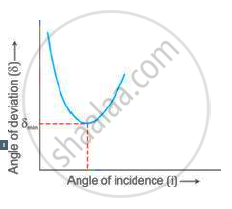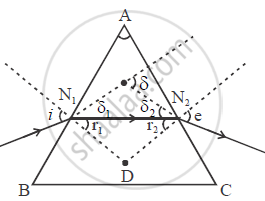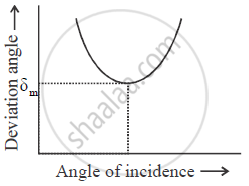# How Does the Angle of Deviation Produced by a Prism Change with Increase in the Angle of Incidence. Draw a Curve Showing the Variation in the Angle of Deviation with - Physics

How does the angle of deviation produced by a prism change with increase in the angle of incidence. Draw a curve showing the variation in the angle of deviation with the angle of incidence at a prism surface.

#### Solution 1

As the angle of incidence increases, the angle of deviation decreases first and reaches to a minimum value (δm) for a certain angle of incidence. By further increasing the angle of incidence, the angle of deviation is found to increase.
Variation of angle of deviation (δ) with angle of incidence(i):#### Solution 2

The deviation angle first increases with increasing incidence angle and then decreases. From figure,

δ = δ1 + δ2                               ....(i)

and i = δ11 ; e =δ2

⇒ i = γ1 = δ                     ....(ii)

⇒ e - γ1 = δ2                           ....(iii)

from ΔON1 N

γ1 +γ+ ∠N1 ON2=180°            ....(iv)

∠A + ∠N1ON2 = 180°                 ....(v)

from (iv) and (v)

γ1 +γ2 = A

δ = i - γ1 + e - γ2

=( i - e ) -( γ1 + γ2)                    ...(vi)

at δm

i = e ; γ1 = γ2= γ

δm =2i - A                                          ..(vii)The variation in the angle of deviation with the angle of incidence at a prism surfaceGraph between i and  δ

Concept: Refraction of Light Through a Rectangular Glass Slab
Is there an error in this question or solution?

#### APPEARS IN

Selina Concise Physics Class 10 ICSE
Chapter 4 Refraction of Light at Plane Surfaces
Exercise 4 (B) | Q 6.1 | Page 87